# Import/Export CSV Files in Pandas MCQ | CSV File MCQ

1. Csv stands for

a. Compact serial values
b. Common standard values
c. Control space values
d. Comma separated values

d. Comma separated values

1. Tabular data that saved as plain text where data values are separated by commas

a. Dataframe
b. CSV
c. MySQL
d. All of the above

b, CSV

3. Look at this image and identify the file type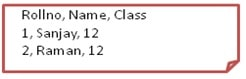a. MySQL
b. Dataframe
c. CSV
d. Excel

c. CSV

1. Read following statement about features of CSV file and select which statement is TRUE?

Statement1: Only database can support import/export to CSV format
Statement2: CSV file can be created and edited using any text editor
Statement3: All the columns of CSV file can be separated by comma ‘ , ‘ only

a. Statement 1 and statement 2
b. Statement 2 and statement 3
c. Statement 2
d. Statement 3

c. Statement 2
STATE1: import/export can be supported by spreadsheet/database/dataframe
STATE3: any delimeter can be used

1. Select the INCORRECT statement

a. CSV files occupies less memory space
b. CSV files are easy to read and write manually
c. CSV files are compatible only with spreadsheet
d. Data value of CSV files can be separated by any delimiter like comma/semicolon/space/tab.

c. CSV files are compatible only with spreadsheet

1. All individual rows of CSV files are called

a. Tuple
b. Data
c. Record
d. Cardinality

c. Record

1. CSV files are text files

a. True
b. False

a. True

1. CSV file can also be considered as Binary files

a. True
b. False
c. Sometimes
d. Not sure

b. False

1. Separator characters used for separating values of CSV files are called

a. comma
b. Delimiter
c. Parameter
d. Spacer

b. Delimiter

1. To read data from CSV file in pandas dataframe  _______ method is used.

b. to_csv()
d. writer()

1. ________ method saves data of dataframe to CSV file.

b. to_csv()
d. writer()

b. to_csv()

12. look at the anatomy of CSV file and select correct abbreviation for 1 and 2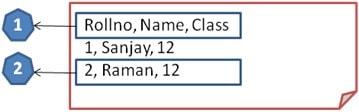a. Attributes, 2. Rows
b. Columns, 2. Data
c. Degree, 2. Record

1. Which of the following is not a parameter (argument) of read_csv() method?
a. sep
b. names
d. sort

d. sort

1. which of the following is not relavent to CSV files?

a. Smaller in size
c. Easy to parse
d. Test and numeric data are distinct

d. Test and numeric data are distinct

1. Raju want to make a dataframe ‘saledata’ based on data of sale.csv which is stored in folder ‘Python’ in C: drive. What code he should write for it?

c. Saledata = pd.to_csv(“C:/Python/sale.csv”)
d. All of the above

1. Default delimeter in CSV is

a. ;
b. :
c. I
d. ,

d ,

1. Consider following statement regarding read_csv() and select True Statement(s):
Statement1: bydefault, read_csv() use the value of first row of CSV as column headers in dataframe.
Statement2: we can exclusively specify column names using the parameter ‘names’ while creating dataframe using read_csv()
Statement3: we cannot import selective records/rows in dataframe while creating using read_csv()

a. Statement1, statment2
b. Statement1, statement3
c. Statement2, statement3
d. All are true

a. Statement1, statment2

1. (i)_______ attribute used with read_csv() to import selective records/rows in dataframe
(ii)________ attribute used with read_csv() to specify the number of row whose values are to be used as column names.

a. (i) nrows, (ii) names
b. (i) rows, (ii) pos

1. Attribute used to specify the separator character for values being imported in dataframe using read_csv()

a. Sepatator
b. Sep
c. Sepies
d. Space

b. Sep

1. Write code to read data from CSV file student.csv stored in C: in dataframe ‘std’ including exculsive columns rollno, name, percent where all values are separated by semicolon ‘:’.

a. Std = pd.read_csv(“C:\student.csv”, sep = ‘;’, names = [‘rollno’,’name’,’percent’])
b. Std = pd.read_csv(“C:\student.csv”, sepies = ‘;’, names = (‘rollno’,’name’,’percent’))
d. Std = pd.read_csv(“C:\student.csv”, sepies = ‘;’, columns = [‘rollno’,’name’,’percent’])

a. Std = pd.read_csv(“C:\student.csv”, sep = ‘;’, names = [‘rollno’,’name’,’percent’])

1. Raju want to import first 5 records of all items of stock.csv stored in ‘inventory’ folder of c frive in dataframe ‘product’. Help him to write correct statement.

b. Product = pd.read_csv(“c:\inventory\stock.csv”, rows = 5)
c. Product = pd.read_csv(“c:\inventory\stock.csv”, nrows = 5)
d. Product = pd.read_csv(“c:\inventory\stock.csv”, top = 5)

c. Product = pd.read_csv(“c:\inventory\stock.csv”, nrows = 5)

22. For given sale.CSV located in C:\invetory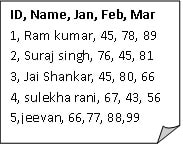Shivangi want to create dataframe ‘Sales’ as given below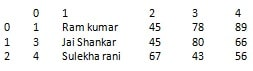Help her to write correct code to do so.

c. Sales = pd.read_csv(“c:\inventory\sale.csv”, columns =[0,1,2,3,4], skiprows = [2,5])

1. A CSV file can take __________ as delimeter

a. ;
b. |
c. \t
d. @
e. All of the above

e. All of the above

1. Write a program to read from CSV file “c:\python\data.csv” where the delimeter is @ with following conditions
(i) Give exclusive column names as col1, col2, col3
(ii) First row should be taken as data and not as column headers

1. Which of the following attribute should be taken to import first 10 rows in a dataframe from CSV?

a. Nrows = 10
b. Rows = 10
c. Skiprows = 10

a. Nrows = 10

26. To create dataframe T from following csv file temp.csv stored in c drive, which of the following code is correct?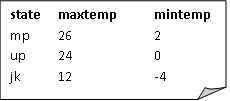b. T = pd.read_csv(“c:\temp.csv”,sep = “ “)
c. T = pd.read_csv(“c:\temp.csv”, sep = “\n”)
d. T = pd.read_csv(“c:\temp.csv”, sep = “\t”)

d. T = pd.read_csv(“c:\temp.csv”, sep = “\t”)

1. Which of the following statement is INCORRECT regarding to_csv() method?
Statement1: We cannot export specified columns of a dataframe to CSV files
Statement2: We can exclude column names to be saved to CSV file which exporting using header = none
Statement3: We can exclude row labels of dataframe to be written in CSV file while exporting using index = False

a. Statement1, statment2
b. Statement2, statement3
c. Statement1, statement3
d. Statement2

a. Statement1, statment2
Statement1: We can export specified columns of a dataframe to CSV files using columns
Statement2: We can exclude column names to be saved to CSV file which exporting using header = False

1. Select appropriate code to export all data of dataframe df to csv file temp.csv located in c drive excluding row labels.

a. df.to_csv(“C:\temp.csv”)
b. df.to_csv(“C:\temp.csv”, index = False)
d. None of the above

b. df.to_csv(“C:\temp.csv”, index = False)

1. For given dataframe book

Create csv file lib.csv with columns bname and price.

a. Book.to_csv(“lib.csv”, columns = (‘bname’,’price’))
b. Book.to_csv(“lib.csv”, columns = [‘bname’,’price’])
c. Book.to_csv(“lib.csv”, col = [‘bname’,’price’])
d. Book.to_csv(“lib.csv”, index_col = [‘bname’,’price’])

b. Book.to_csv(“lib.csv”, columns = [‘bname’,’price’])

1. For given dataframe ‘res’

Somesh has written following code to create marks.csv
Res.to_csv(“c:\result\marks.csv”,index = False, header = False, Columns = [‘Hindi’,’Sanskrit’])

Predict the output

```a.
5    9    10
8    6    8
5    6    7

b.
5    10
8    8
5    7

c.
0    1    2
0    5    9    10
1    8    6    8
2    5    6    7

d. Error```

```b.
5    10
8    8
5    7```

1. If you want to read top 20 rows of data from CSV file, which argument would you give to read_csv()?

a. Rows
b. Nrows

b. Nrows

1. By default, read_csv() uses the value of first row as column header in dataframes. Which argument will you give to ensure that the top/first row’s data is used as data and not as column header?

d. Skiprows= 1

1. If you do not want to include index labels of dataframe being exported to CSV files, which argument will you give to ensure that?

a. Axes = False
b. Index  =  False
c. Index  = None
d. Axes=None

b. Index  =  False

1. If you do not want the column names to be saved to the csv file, we may use

1.